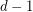# A generalization of Vizing's Theorem?

 Importance: Medium ✭✭
 Author(s): Rosenfeld, Moshe
 Subject: Graph Theory » Coloring » » Edge coloring
 Keywords: edge-coloring hypergraph Vizing
 Posted by: mdevos on: April 11th, 2007
Conjecture   Letbe a simple-uniform hypergraph, and assume that every set ofpoints is contained in at mostedges. Then there exists an-edge-coloring so that any two edges which sharevertices have distinct colors.

Vizing's Theorem is equivalent to the above statement for. For higher dimensions, this problem looks difficult since the main tool used in the proof of Vizing's theorem (Kempe chains) do not appear to work.

### Reference

Could someone please add a reference? There should be some paper (or a conference talk?) where Rosenfeld proposed the conjecture.

-DOT